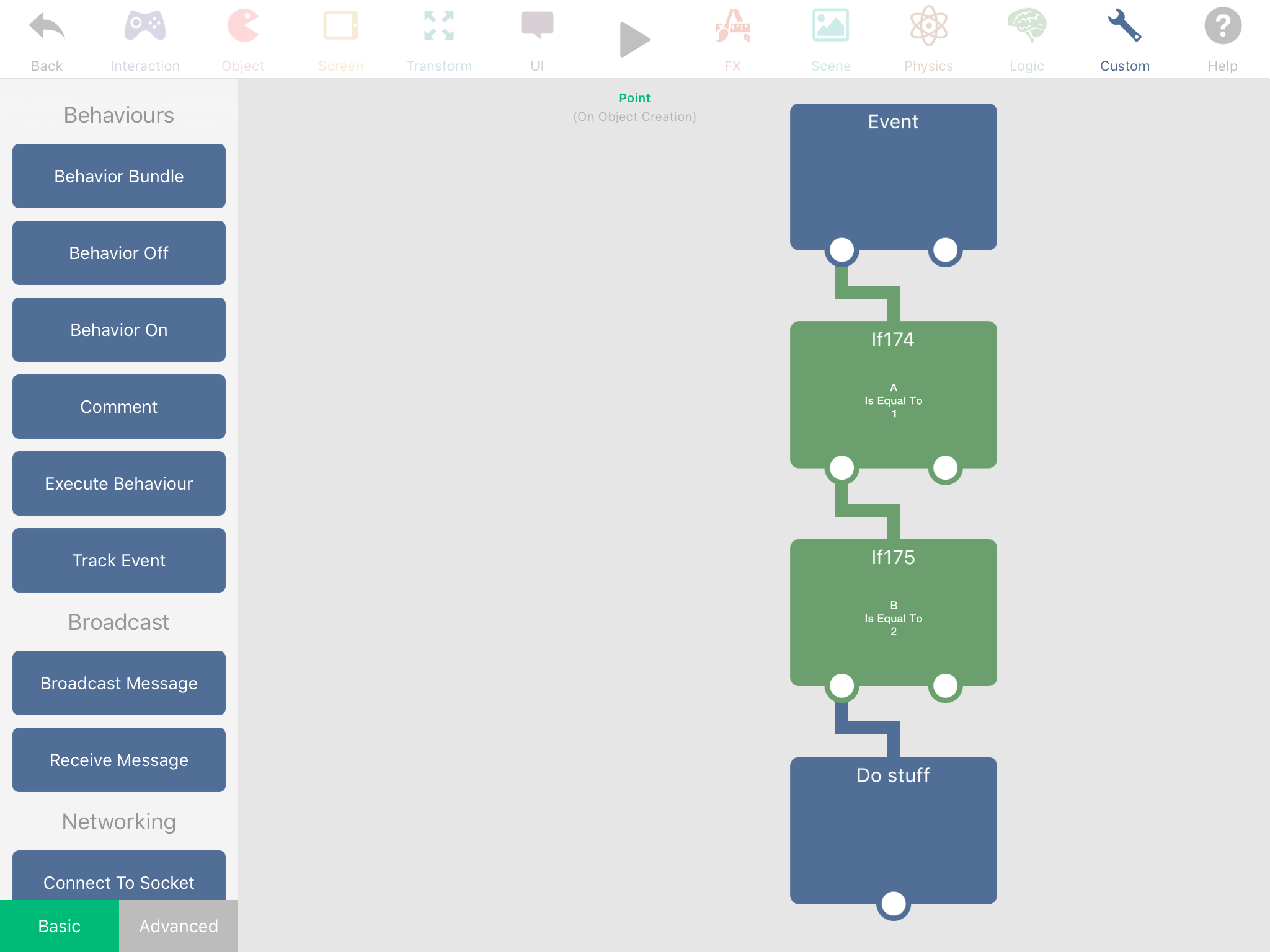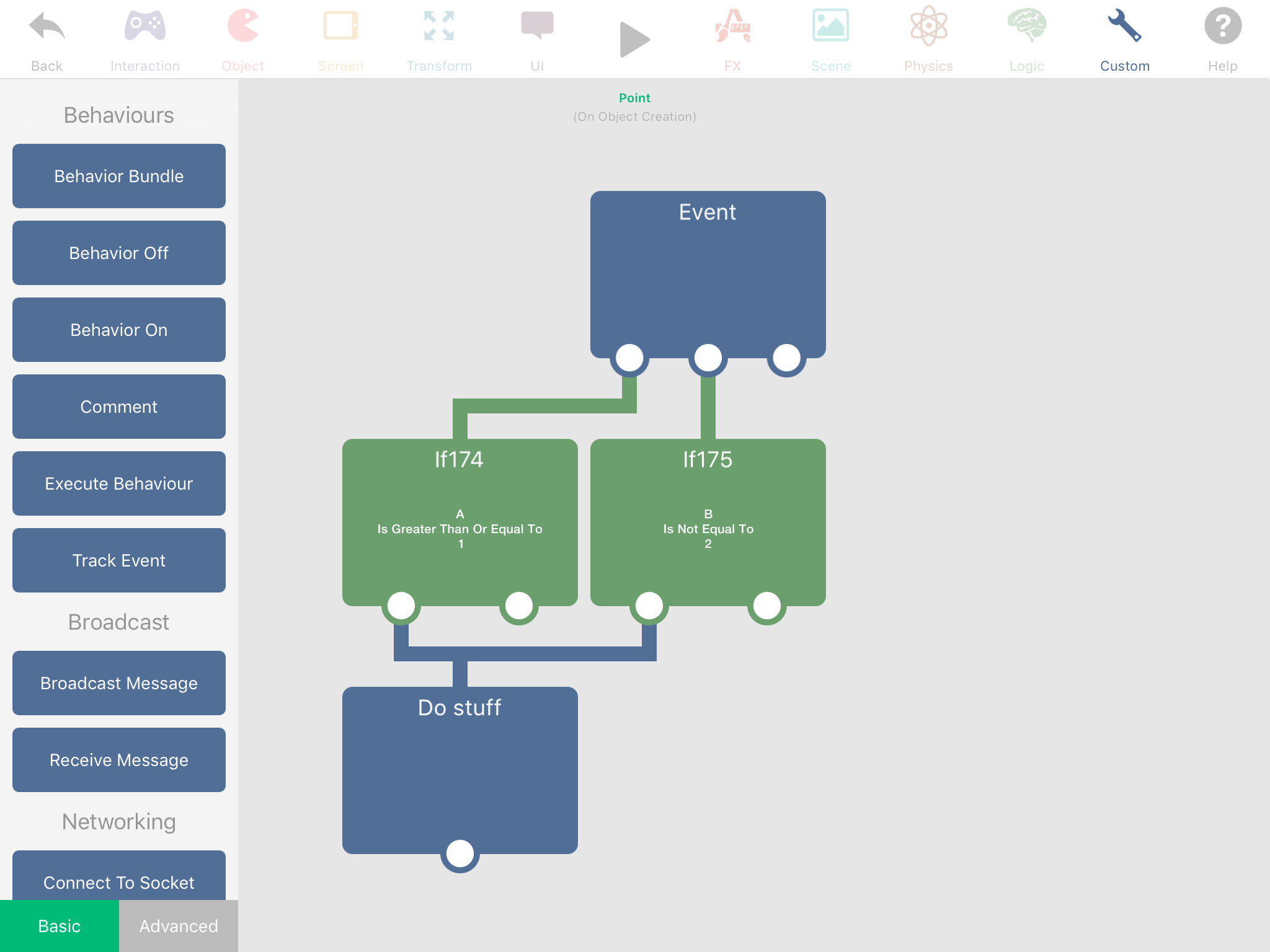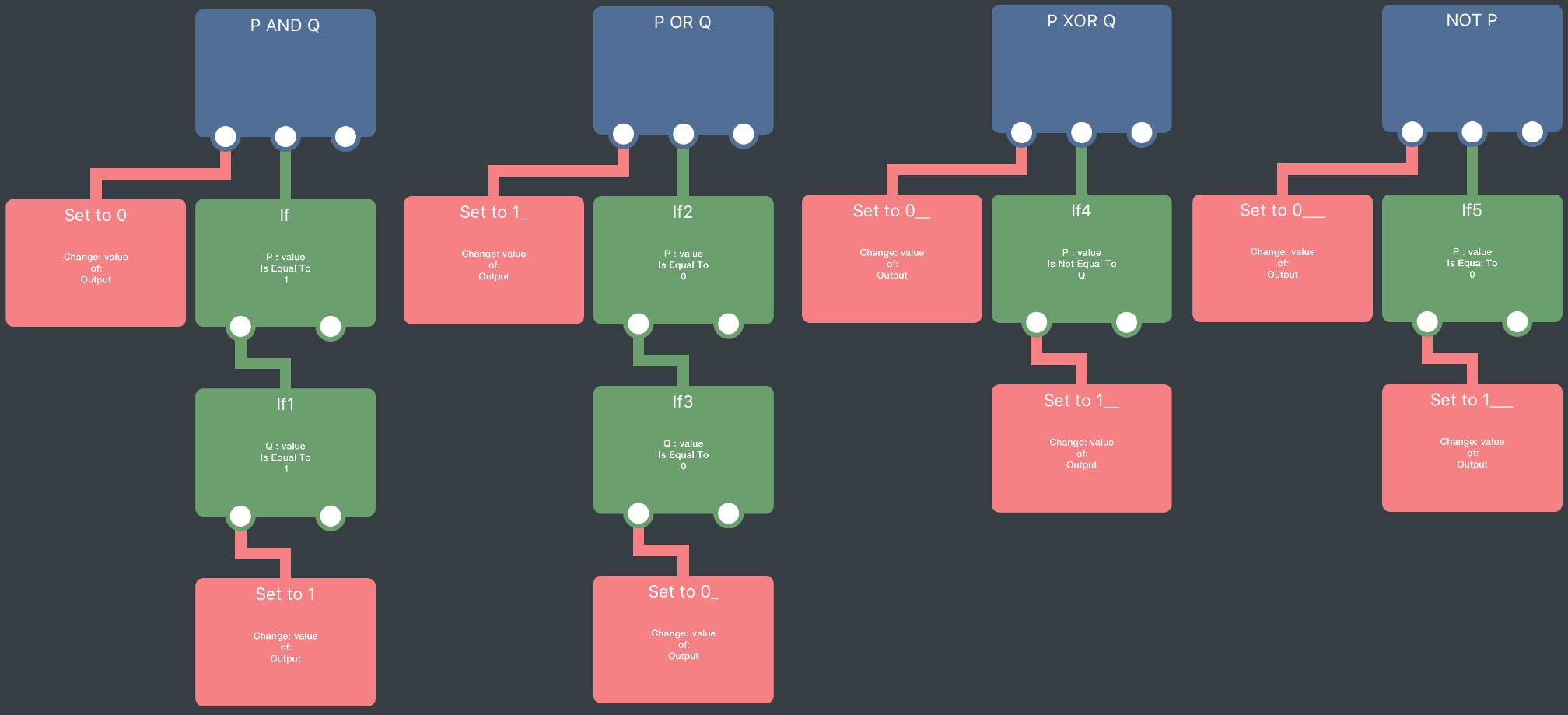# How to do logical AND in conditionals?

• If this AND that then jump

... how to do this in hyperPad?

• @Deeeds Have an If stacked on top of another If. The more conditions you have, the longer your If chain is.

•• @Aidan-Oxley What about OR, XOR and NOT?

• @Deeeds For Or, have the two Ifs next to each other underneath the event. I don’t know what XOR is. For not, press the “is equal to” when you tap the If behaviour, there will be a not equal to (≠).

•• @Deeeds

###### (I'm going to use this to learn the code formatting on the forums)

Like Aidan said, (P AND Q) can be represented by nesting ifs. It can be done as

``````//# I'm not sure I fully get the syntax for comments
output = 0 //# default to 0 if they aren't both true
if P == 1:
if Q == 1
output = 1 //# set to 1 if they are both true
return output
``````

P OR Q:

``````output = 1 //# defaults to 1
if P == 0
if Q == 0
output = 0 //# if NOT(P) AND NOT(Q) is true, return 0
return output
``````

(P XOR Q) is the equivalent to ((P OR Q) AND NOT(P AND Q)), but there's an easy shortcut in that it's true when P ≠ Q:

``````output = 0
if P != Q:
output = 1
return output
``````

Edit: oh yeah, NOT P:

``````output = 1
if P = 1
output = 0
return output
``````